Download Presentation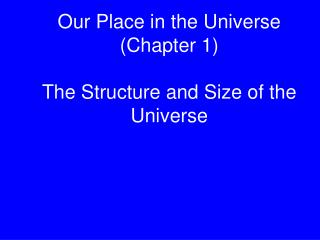Our Place in the Universe (Chapter 1) The Structure and Size of the Universe

# Our Place in the Universe (Chapter 1) The Structure and Size of the Universe - PowerPoint PPT Presentation

Our Place in the Universe (Chapter 1) The Structure and Size of the Universe. Based on Chapter 1. This material will be useful for understanding Chapters 2, 3, and 13 on “Years, Seasons, and Months”, “The Orbits of the Planets”, and “Extrasolar Planets”. Goals for Learning.I am the owner, or an agent authorized to act on behalf of the owner, of the copyrighted work described.
Download Presentation## Our Place in the Universe (Chapter 1) The Structure and Size of the Universe

An Image/Link below is provided (as is) to download presentation

Download Policy: Content on the Website is provided to you AS IS for your information and personal use and may not be sold / licensed / shared on other websites without getting consent from its author.While downloading, if for some reason you are not able to download a presentation, the publisher may have deleted the file from their server.

- - - - - - - - - - - - - - - - - - - - - - - - - - E N D - - - - - - - - - - - - - - - - - - - - - - - - - -
Presentation Transcript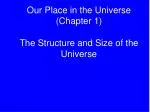### Our Place in the Universe (Chapter 1)The Structure and Size of the Universe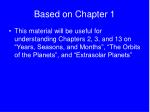Based on Chapter 1
• This material will be useful for understanding Chapters 2, 3, and 13 on “Years, Seasons, and Months”, “The Orbits of the Planets”, and “Extrasolar Planets”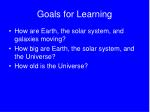Goals for Learning

• How are Earth, the solar system, and galaxies moving?
• How big are Earth, the solar system, and the Universe?
• How old is the Universe?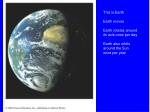This is Earth

Earth moves

Earth rotates around

its axis once per day

Earth also orbits

around the Sun

once per year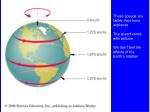These speeds are

faster than most

airplanes

The speed varies

with latitude

We don’t feel the

effects of the

Earth’s rotation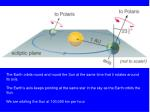The Earth orbits round and round the Sun at the same time that it rotates around

its axis.

The Earth’s axis keeps pointing at the same star in the sky as the Earth orbits the

Sun.

We are orbiting the Sun at 100,000 km per hour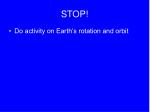STOP!
• Do activity on Earth’s rotation and orbit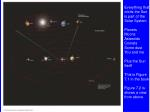Everything that

orbits the Sun

is part of the

Solar System

Planets

Moons

Asteroids

Comets

Some dust

You and me

Plus the Sun

Itself

This is Figure

7.1 in the book

Figure 7.2 is

shows a view

from above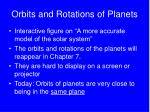Orbits and Rotations of Planets
• Interactive figure on “A more accurate model of the solar system”
• The orbits and rotations of the planets will reappear in Chapter 7.
• They are hard to display on a screen or projector
• Today: Orbits of planets are very close to being in the same planeA star shines with its

own light.

The Sun is a star.

The Moon only reflects

light from the Sun, so

it is not a star.

Stars are large balls of

hot gas, mostly

hydrogen and helium

The Sun generates

heat and light by a

process called nuclear

fusion

This is different from

what happens in nuclear

power stationsStars form groups called galaxies

This is a fake picture of our galaxy,

the Milky Way

It contains 100 billion starsEvery object in this picture is a galaxy that contains billions of stars.

The Universe contains at least 100 billion galaxiesAstronomers can measure how

far away galaxies are and how

fast they are moving

All the galaxies in the Universe,

including our Milky Way, are

spreading apart and moving

away from each other.

Galaxies that are close together

are moving apart slowly, but

galaxies that are far apart are

moving apart more quickly.

How can this happen? The

entire Universe is, for some

reason, expanding.

Galaxies themselves are not

getting larger, but the distances

between galaxies are increasing

Do raisin loaf demo (fig 1.16)Summary
• Earth rotates around its axis
• Earth also orbits around the Sun
• The Sun is one of many stars in our galaxy, the Milky Way
• The Milky Way is one of many galaxies in the Universe
• All galaxies in the Universe are moving away from each other – the Universe is expandingPowers of Ten
• 0.000000000000000000001 is a very small number
• 10000000000000000000000 is a very large number
• We will discuss very big things, like the Universe, and very small things, like atoms
• We need a way to write these kinds of numbers more compactlyPowers of Ten
• Rule 1: 101 = 10
• Rule 2: 10A x 10B = 10(A+B)
• 10 x 10 = 100
• 10 x 10 = ?Powers of Ten
• Rule 1: 101 = 10
• Rule 2: 10A x 10B = 10(A+B)
• 10 x 10 = 100
• 10 x 10 = 101 x 101 = ?Powers of Ten
• Rule 1: 101 = 10
• Rule 2: 10A x 10B = 10(A+B)
• 10 x 10 = 100
• 10 x 10 = 101 x 101 = 10(1+1) = ?Powers of Ten
• Rule 1: 101 = 10
• Rule 2: 10A x 10B = 10(A+B)
• 10 x 10 = 100
• 10 x 10 = 101 x 101 = 10(1+1) = 102
• So 102 = 100Some Exercises
• 103 = ?
• 10000 = ? (Give answer as Power of Ten)
• 100 x 102 = ? (Give answer as Power of Ten)
• 104 x 104 x 1019 = ? (Give answer as Power of Ten)Division
• 104 / 103 = ?
• 104 / 102 = ?
• 104 / 101 = ?
• 10A / 10B = 10?Division
• 104 / 103 = 10 = 101
• 104 / 102 = 100 = 102
• 104 / 101 = 1000 = 103
• 10A / 10B = 10(A-B)What is 100 ?
• 10A x 10B = 10(A+B)
• 101 x 100 = 10(1+0) = 101
• 101 x ? = 101
• 10 x ? = 10What is 10-1?
• 10A / 10B = 10(A-B)
• 100 / 101 = 10-1
• 1 / 10 = 10-1
• 0.1 = 10-1Some Exercises
• 0.1 x 0.1 = ? (Give answer as Power of Ten)
• 0.1 x 0.1 x 0.1 = ? (Give answer as Power of Ten)
• 1 / 106 = ? (Give answer as Power of Ten)
• 102 / 104 = ? (Give answer as Power of Ten)Summary
• Rule 1: 101 = 10
• Rule 2: 10A x 10B = 10(A+B)
• 102 = 10 x 10 = 100
• 101 = 10
• 100 = 1
• 10-1 = 0.1
• 10-2 = 0.1 x 0.1 = 0.01The Age of the Universe
• The Universe is very old
• Astronomers know how far away other galaxies are
• Astronomers know how quickly those galaxies are moving away from us
• By comparing those distances and speeds, they can estimate how long the Universe has been expanding for
• 14 billion years – The age of the Universe
• The Solar System is younger. It only formed 4.5 billion years ago

Interactive Raisin LoafThe Size of the Universe
• Astronomers don’t know the size of the Universe.
• We’ve never observed anything like the edge of the Universe
• Without any edges, there can’t be a “centre of the Universe”
• Astronomers do know that the Universe must be larger than a certain size…The Speed of Light

Light travels very quickly, much faster than anything else in our everyday lives.

However, the Universe is so big that it takes a long time for light to travel from one

galaxy to another. It takes 2.5 million years for light from the nearby Andromeda

Galaxy to reach us.

If you look at the Andromeda Galaxy today, you see it as it looked

2.5 million years ago.Light-Years
• Light travels at 300,000 km per second
• Fast enough to circle Earth 8 times every second
• 1 Light-year = the distance that light can travel in 1 year
• 1 light-year is about 10,000,000,000,000 km or 1013 kmLooking Back in Time

When we look at the sky, we see nearby objects as they were a short time ago, but we

see far-away objects as they were a long time ago

We can’t see anything beyond 14 billion light years away from Earth, but that doesn’t

mean that nothing exists that far awaySizes of Things
• Universe – 14 billion (14 x 109) light years or more
• Galaxy – 105 light years
• Distances between galaxies varies a lot, but can be around 100x the typical diameter of a galaxy
• There is plenty of empty space between galaxiesSizes of Things
• Galaxy – 105 light years
• Closest star to the Sun – 4 light years or 4 x 1013 km
• Distance from the Sun to Pluto – 0.006 light years = 6 x 109 km
• Diameter of the Sun – 7 x 105 km
• You could fit 108Suns in the distance between the Sun and its closest neighbour
• There is a lot of empty space between stars (or between stellar systems) in galaxiesSizes of Things
• Distance from the Sun to Earth – 1.5 x 108 km = 1 Astronomical Unit or 1 AU
• Diameter of the Sun – 7 x 105 km
• Diameter of the Earth – 6400 km
• There is a lot of empty space in the Solar System between the Sun and its planetsGoals for Learning
• How are Earth, the solar system, and galaxies moving?
• How big are Earth, the solar system, and the Universe?
• How old is the Universe?Goals for Learning
• How are Earth, the solar system, and galaxies moving?
• Earth rotates on its axis once per day and orbits the Sun once each year. Earth’s axis is tilted from its orbital plane
• Everything in the solar system orbits the Sun. The Sun orbits the centre of the Milky Way
• Galaxies are receding from each other, so the Universe is expandingGoals for Learning
• How big are Earth, the solar system, and the Universe?
• Earth is 6400 km radius and 1.5x108 km from the Sun
• The solar system is about 100 AU radius
• Typical galaxies contain 100 billion stars and the Universe contains 100 billion galaxies
• The Universe is at least 14 billion light years acrossGoals for Learning
• How old is the Universe?
• The Universe formed 14 billion years ago in the Big Bang
• The solar system formed 4.5 billion years agoCompressed History
• The Universe is 14 billion years old. If the history of the Universe were compressed into 1 year, this is what the calendar would look like.
• 14 billion years -> 1 compressed year
• 1.2 billion years -> 1 compressed month
• 270 million years -> 1 compressed week
• 38 million years -> 1 compressed day
• 1.6 million years -> 1 compressed hour
• 27,000 years -> 1 compressed minute
• 440 years -> 1 compressed secondHow far is a light-year?
• The Milky Way Galaxy is 100,000 light years across. The nearest star to the Sun, Alpha Centauri, is 4 light years away. 1 light-year equals 1013 km.
• If you had a ruler whose length was one of these distances, how many times would you need to lay the ruler end-to-end before you had measured out one light-year?
• Sun - Pluto distance = 6 x 109 km
• Sun - Earth distance = 1.5 x 108 km
• Sun’s diameter = 1.4 x 106 km
• Earth - Moon distance = 3.8 x 105 km
• Earth’s diameter = 1.2 x 104 km